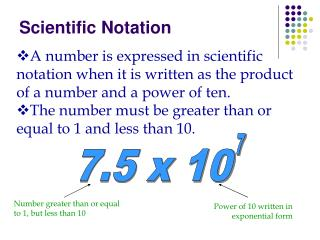DownloadDownload PresentationScientific Notation

# Scientific Notation

Download Presentation## Scientific Notation

- - - - - - - - - - - - - - - - - - - - - - - - - - - E N D - - - - - - - - - - - - - - - - - - - - - - - - - - -
##### Presentation Transcript

1. 7 7.5 x 10 Scientific Notation • A number is expressed in scientific notation when it is written as the product of a number and a power of ten. • The number must be greater than or equal to 1 and less than 10. Number greater than or equal to 1, but less than 10 Power of 10 written in exponential form

2. The Distance From the Sun to the Earth 93,000,000

3. Step 1 • Move decimal to the left • Leave only ONE number in front of decimal 93,000,000 --> 9.3000000

4. Step 2 • Write number without zeros 9.3000000 = 9.3

5. 7 93,000,000 = 9.3 x 10 Step 3 • Count how many places you moved the decimal • Make that your exponent

6. 9.85 x 107 -----> 6.41 x 1010 -----> 2.79 x 108 -----> 4.2 x 105 -----> Practice Problems Write in scientific notation. Decide the exponent. • 98,500,000 = 9.85 x 10? • 64,100,000,000 = 6.41 x 10? • 279,000,000 = 2.79 x 10? • 420,000= 4.2 x 10?

7. Standard form is the opposite of scientific notation • To write in standard form, move the decimal the same number of places as the exponent. • The sign of the exponent tells which direction to move the decimal. A positive exponent means move the decimal to the right.

8. More Practice Problems Write in standard form • 7.34 x 108 • 8.7 x 103 • 9 x 105 Answers • 734,000,000 3) 900,000 2)8,700

9. Let’s get started on the homework • W.S. 3-7 Front AND back • Remember to be quiet!!

10. Warm-Up Write in scientific notation 1.) 2,000,000,000 2.) 36,500,000 3.) 465,000,000,000,000 Write in standard form 4.) 4.62 x 105 5.) 3 x 103

11. What if your number is a decimal? Step 1: • Move decimal to the right • Leave only one number in front of decimal 0.0000896 --> 00008.96

12. Step 2 • Write number without zeros 000008.96 = 8.96

13. -5 0.0000896 = 8.96 x 10 Step 3 • Count how many places you moved the decimal • Make that your exponent • Since your number was a decimal to begin with the power is a negative!

14. 6.58 x 10-6 -----> 6.41 x 10-10 -----> 1.23 x 10-8 -----> 6.8 x 10-6 -----> Practice Problems Write in scientific notation. Decide the power of ten. • 0.00000658 = 6.58 x 10? • 0.000000000641 = 6.41 x 10? • 0.0000000123 = 1.23 x 10? • 0.0000068 = 6.8 x 10?

15. Standard form is the opposite of scientific notation • To write in standard form, move the decimal the same number of places as the exponent. • The sign of the exponent tells which direction to move the decimal. A positive exponent means move the decimal to the right,and a negative exponent means move the decimal to the left.

16. More Practice Problems Write in standard form • 4.35 x 10-8 • 8.7 x 10-6 • 5.2 x 10-5 Answers • 0.0000000435 2)0.0000087 3) 0.000052